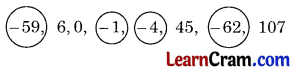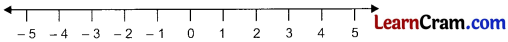# DAV Class 6 Maths Chapter 3 Worksheet 1 Solutions

The DAV Class 6 Maths Book Solutions Pdf and DAV Class 6 Maths Chapter 3 Worksheet 1 Solutions of Integers offer comprehensive answers to textbook questions.

## DAV Class 6 Maths Ch 3 WS 1 Solutions

Question 1.
Indicate the following by using integers.
(a) Earning of ₹ 500
Solution:
+ 500

(b) Loss of ₹ 90
Solution:
– 90

(c) Climbing up 10 steps
Solution:
+ 10

(d) Withdrawal of ₹ 500 from a bank
Solution:
– 500

(e) 5 m above sea level
Solution:
+ 5

(f) 3 km towards north
Solution:
+ 3

(g) 10° C below zero
Solution:
– 10

(h) An increase of 25 marks
Solution:
+ 25Question 2.
Write the opposite of:

(a) Depositing ₹ 1000 in a bank account.
Solution:
Withdrawal of 1000 from a bank account.

(b) Decrease of 5 marks.
Solution:
Increase of 5 marks.

(c) Earning ₹ 200.
Solution:
Losing of ₹ 200.

(d) Going 2 km towards east.
Solution:
Going 2 km towards west.

(e) Two steps to the left of zero on number line.
Solution:
Two steps to the right of zero on number line.

(f) Losing weight of 7 kg.
Solution:
Gaining weight of 7 kg.

Question 3.
Encircle the negative integers from the following numbers.
– 59, 6, 0, – 1, – 4, 45, – 62, 107
Solution:### DAV Class 6 Maths Chapter 3 Worksheet 1 Notes

All positive, zero and all negative numbers are called Integers.
Example:
………, – 5, – 4, – 3, – 2, – 1, 0, 1, 2, 3, 4, 5, ………

Number Line:Absolute Value:
The absolute value of an integer is its numerical value having no (+) and (-) sign.
Example:
| 2 | = 2 and | – 2 | = 2

• + 2 + 3 = + 5
• (+ 2) + (- 3) = – 1
• (4) + (- 5) = – 9
• (- 3) + (+ 4) = 1

Subtraction of Integers:

• (+ 2) – (+ 6) = – 4
• (+ 3) – (- 4) = – 3 + 4 = 1
• (- 5) – (+ 7)= 2
• (+ 4) – (+ 6) = – 2Multiplication of Integers:

• (+ 5) × (+ 3) = 15
• (- 5) × (3)= – 15
• (+ 5) × (- 3) = – 15
• (- 5) × (- 3) = 15

Division of Integers:

• (+ 6) ÷ (+ 2) = 3
• (+ 6) ÷ – 2) = – 3
• (- 6) ÷ (+ 2) = – 3
• (- 6) ÷ (- 2) = 3

Power of Integers:

• (+ 3)2 = (+ 3) × (- 3) = 9
• (- 3)2 = (- 3) × (- 3) = 9